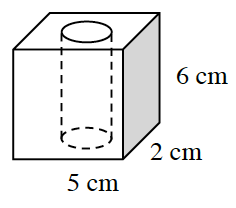### Home > CCG > Chapter 10 > Lesson 10.1.1 > Problem10-6

10-6.A rectangular prism has a cylindrical hole removed, as shown at right.

1. If the length of the radius of the cylindrical hole is $0.5$ cm, find the volume of the solid.

$\text{Volume of cube}-\text{volume of cylinder}=\text{volume of solid}$

$\text{Volume of cylinder}=\pi r^2h\\ v=\pi\left(0.5\right)^2\left(6\right)\\ v=1.5\pi\approx4.71\ \text{cm}^3$

$\text{Volume of cube}=l\cdot w\cdot h\\ v=5\left(2\right)\left(6\right)=60\ \text{cm}^3$

$\text{Volume of cube}-\text{volume of cylinder}=60\ \text{cm}^3-4.71\ \text{cm}^3$

$≈ 55.3\text{ cm}³$

2. What could this geometric figure represent? That is, if it were a model for something that exists in the world, what might it be? Also, how might you change it to make it a better model?